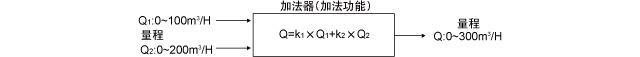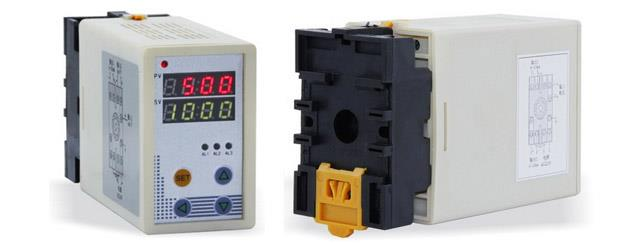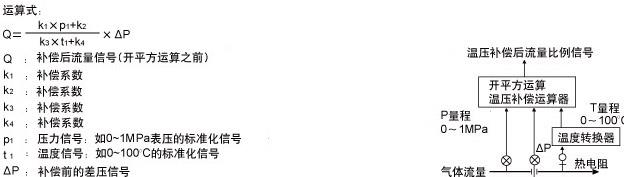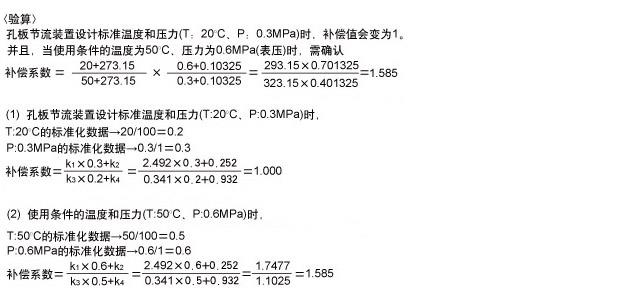﻿ 控制系统使用的数学运算器如何设置系数

# 控制系统使用的数学运算器如何设置系数

2016/8/21 18:55:42 人评论 次浏览 分类：过程控制  文章地址：http://yunrun.com.cn/tech/658.html<验算> 对Q1为50m³/H、Q2为150m³/H时，Q将变为200m³/H进行确认。

Q1的标准化数据: 50÷100=0.5
Q2的标准化数据: 150÷200=0.75
Q的标准化数据: k1×Q1的标准化数据+k2×Q2的标准化数据
=0.333×0.5+0.667×0.75=0.167+0.5=0.667<验算> 当Q1为50m³/H时，如果将比值设定器的刻度设置为2(实际信号比为1)，确认FIC的设定值SV将变为100m³/H。

FIC的设定值=Q1的标准化数据×比值设定器的实际信号比=0.5×1=0.5
Q2的流量将变为0.5×200=100m³/H。k1: (压力量程的最大值-压力量程的最小值)/(设计标准压力+大气压)
=(1-0)/(0.3+0.101325)=1/0.401325=2.492
k2: (压力量程的最小值+大气压)/(设计标准压力+大气压)
=(0+0.101325)/(0.3+0.101325)=0.101325/0.401325=0.2525
k3: 温度量程的最大值-温度量程的最小值)/(设计标准温度+273.15)
=(100-0)/(20+273.15)=100/293.15=0.341
k4: (温度量程的最小值+273.15)/(设计标准温度+273.15)
=(0+273.15)/(20+273.15)=273.15/293.15=0.932与在线工程师QQ交流 到论坛与网友交流 向昌晖仪表网投稿 免费获得2000元电子书

客户姓名：